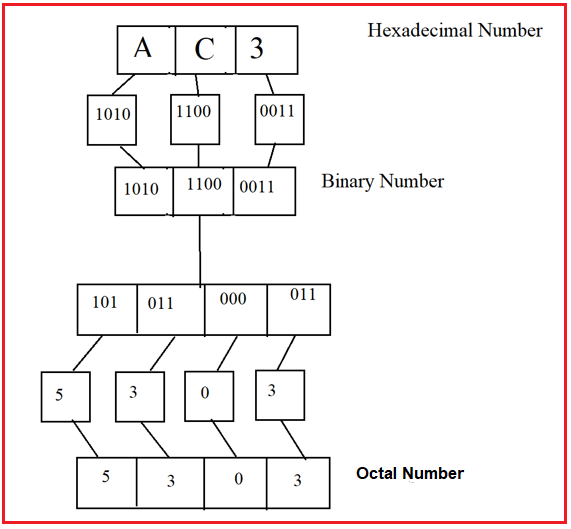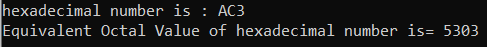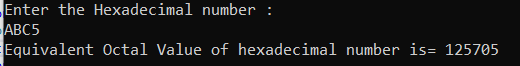# Hexadecimal to Octal Conversion in C#

## Hexadecimal to Octal Conversion in C# with Examples

In this article, I am going to discuss Hexadecimal to Octal Conversion in C# with Examples. Please read our previous article, where we discussed Decimal to Octal Conversion in C#. At the end of this article, you will understand, what are Hexadecimal and Octal Number System is and how to convert a Hexadecimal Number into its equivalent Octal Number using C#.

##### What is Hexadecimal Number System?

In the mathematics, Hexadecimal number system is a positional numeral system with a base, of 16. It uses sixteen distinct symbols, most often the symbols 0–9 to represent values zero to nine, and A, B, C, D, E, F (or alternatively a, b, c, d, e, f) to represent values ten to fifteen. Example: (AC3)16

##### What is Octal Number System?

The octal numeral system or oct is the base-8 number system and uses the digits 0 to 7. Octal numerals can be made from binary numerals by grouping consecutive binary digits into groups of three (starting from the right). Example- (5303)8

##### Steps for hexadecimal to Octal conversion

Octal= 5303
The binary value of A: 1010
The binary value of C: 1100
The binary value of 1: 0011

Grouping in terms of 3: 101 011 000 011
The octal value of 101: 5
The octal value of 011: 3
The octal value of 000: 0
The octal value of 011: 3
So, the Octal number is = 5303

##### Logic to Convert Hexadecimal to Octal in C#

To find the conversion of hexadecimal to its equivalent binary number, simply we need to follow the below steps

1. First, we have to find the equivalent binary number for each digit given in hexadecimal number. And add 0’s to the left side if any binary equivalent is shorter than 4 bits.
2. Then we have to separate the binary digits into groups, of 3 bits from right to left.
3. After that get the octal equivalent for each binary group.
4. After all the steps our result will print as output.

For better understanding, please have a look at the following image.##### Example: Hexadecimal to Octal Conversion in C#

The following C# Program will convert a hexadecimal number to its equivalent octal number.

```using System;
class HexaToOctal
{
public static void Main(string[] args)
{
long deci = 0;
long c = hexadecimal.Length - 1;
for (int i = 0; i < hexadecimal.Length; i++)
{
switch (ch)
{
case '0':
case '1':
case '2':
case '3':
case '4':
case '5':
case '6':
case '7':
case '8':
case '9':
deci = deci + Int32.Parse(ch.ToString()) * (int)Math.Pow(16, c);
c--;
break;
case 'a':
case 'A':
deci = deci + 10 * (int)Math.Pow(16, c);
c--;
break;
case 'b':
case 'B':
deci = deci + 11 * (int)Math.Pow(16, c);
c--;
break;
case 'c':
case 'C':
deci = deci + 12 * (int)Math.Pow(16, c);
c--;
break;
case 'd':
case 'D':
deci = deci + 13 * (int)Math.Pow(16, c);
c--;
break;
case 'e':
case 'E':
deci = deci + 14 * (int)Math.Pow(16, c);
c--;
break;
case 'f':
case 'F':
deci = deci + 15 * (int)Math.Pow(16, c);
c--;
break;
default:
Console.Write("Invalid hexa input");
break;
}
}
string octal = "";

while (deci > 0)
{
octal = deci % 8 + octal;
deci = deci / 8;
}
Console.Write("Equivalent Octal Value of hexadecimal number is= " + octal);
}
}
```
###### Output:##### Example: Taking input from the user

In the following C# program, we will take the hexadecimal number from the user and then convert the hexadecimal number to its equivalent octal number and print it on the console.

```using System;
class HexaToOctal
{
public static void Main(string[] args)
{
long deci = 0;
Console.WriteLine("Enter the Hexadecimal number : ");
long c = hexadecimal.Length - 1;
for (int i = 0; i < hexadecimal.Length; i++)
{
switch (ch)
{
case '0':
case '1':
case '2':
case '3':
case '4':
case '5':
case '6':
case '7':
case '8':
case '9':
deci = deci + Int32.Parse(ch.ToString()) * (int)Math.Pow(16, c);
c--;
break;
case 'a':
case 'A':
deci = deci + 10 * (int)Math.Pow(16, c);
c--;
break;
case 'b':
case 'B':
deci = deci + 11 * (int)Math.Pow(16, c);
c--;
break;
case 'c':
case 'C':
deci = deci + 12 * (int)Math.Pow(16, c);
c--;
break;
case 'd':
case 'D':
deci = deci + 13 * (int)Math.Pow(16, c);
c--;
break;
case 'e':
case 'E':
deci = deci + 14 * (int)Math.Pow(16, c);
c--;
break;
case 'f':
case 'F':
deci = deci + 15 * (int)Math.Pow(16, c);
c--;
break;
default:
Console.Write("Invalid hexa input");
break;
}
}
string octal = "";

while (deci > 0)
{
octal = deci % 8 + octal;
deci = deci / 8;
}
Console.Write("Equivalent Octal Value of hexadecimal number is= " + octal);